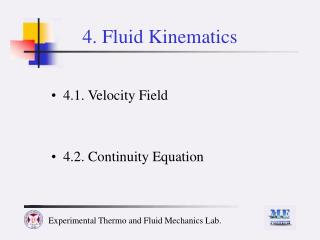Download Presentation4. Fluid Kinematics

# 4. Fluid Kinematics

Download Presentation## 4. Fluid Kinematics

- - - - - - - - - - - - - - - - - - - - - - - - - - - E N D - - - - - - - - - - - - - - - - - - - - - - - - - - -
##### Presentation Transcript

1. 4. Fluid Kinematics • 4.1. Velocity Field • 4.2. Continuity Equation

2. Fluid Kinematics What is fluid kinematics? • Fluid kinematics is the study on fluid motion in space and time without considering the force which causes the fluid motion. • According to the continuum hypothesis the local velocity of fluid is the velocity of an infinitesimally small fluid particle/element at a given instant t. It is generally a continuous function in space and time.

3. How small an how large should be a fluid particle/element in frame of the continuum concept? • The characteristic length of the fluid system of interest >> The characteristic length of a fluid particle/element >> The characteristic spacing between the molecules contained in the volume of the fluid particle/element : • For air at sea-level conditions, • - molecules in a volume of • - (λ : mean free path) • The continuum concept is valid!

4. 4.1. Velocity Field • Eulerian Flow Description • Lagrangian Flow Description • Streamline • Pathline • Streakline

5. 4.1.1. In the Eulerian Method • The flow quantities, like are described as a function of space and time without referring to any individual identity of the fluid particle :

6. 4.1.2. Streamline • A line in the fluid whose tangent is parallel to at a given instant t. • The family of streamlines at time t are solutions of • Where are velocity components in the respective direction

7. Steady flow : the streamlines are fixed in space for all time. • Unsteady flow : the streamlines are changing from instant to instant. ▲ Fig. 4.1

8. 4.1.3. Flow Dimensionality • Most of the real flow are 3-dimensional and unsteady : • For many situations simplifications can be made : 2-dimensional unsteady and steady flow 1-dimensional unsteady and steady flow

9. 4.1.4. In the Lagrangian Method • The flow quantities are described for each individually identifiable fluid particle moving through flow field of interest. The position of the individual fluid particle is a function of time : ▲ Fig. 4.2

10. 4.1.5. Pathline • A line traced by an individual fluid particle : • For a steady flow the pathlines are identical with the streamlines.

11. ▲ Fig. 4.3

12. 4.1.6. Streakline • A streakline consists of all fluid particles in a flow that have previously passed through a common point. Such a line can be produced by continuously injecting marked fluid (smoke in air, or dye in water) at a given location. • For steady flow : The streamline, the pathline, and the streakline are the same.

13. 4.2. Stream-tube and Continuity Equation • Stream-tube • Continuity Equation of a Steady Flow

14. 4.2.1. Stream-tube • is the surface formed instantaneously by all the streamlines that pass through a given closed curve in the fluid. ▲ Fig. 4.4

15. 4.2.2. Continuity Equation of a Steady Flow • For a steady flow the stream-tube formed by a closed curved fixed in space is also fixed in space, and no fluid can penetrate through the stream-tube surface, like a duct wall. ▲ Fig. 4.5

16. Considering a stream-tube of cylindrical cross sections with velocities perpendicular to the cross sections and densities at the respective cross sections and assuming the velocities and densities are constant across the whole cross section , a fluid mass closed between cross section 1 and 2 at an instant t will be moved after a time interval dt by to the cross section 1’ and 2’ respectively. Because the closed mass between 1 and 2 must be the same between 1’ and 2’, and the mass between 1’ and 2 for a steady flow can not change from t and t+dt, the mass between 1 and 1’ moved in dt, must be the same as the mass between 2 and 2’ moved in the same time dt, :

17. Therefore the continuity equation of steady flow : • Interpretation : The mass flow rate • through a steady stream-tube or a duct. • For incompressible fluid with : • Interpretation : The volume flow rate • From the continuity equation for incompressible fluid : • for a stream-tube. (4.1) (4.2)

18. ▲ Fig. 4.6